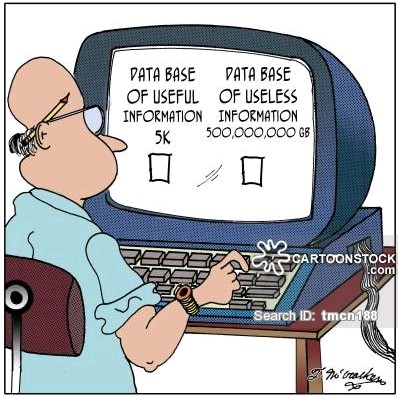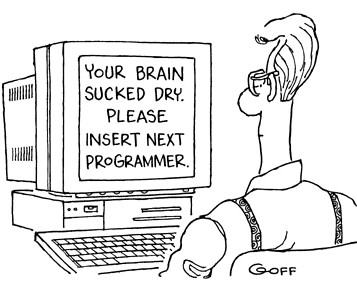## Using Monte Carlo simulations in trading: Key assumptionsLet’s talk about the fundamentals of a Monte Carlo simulation first, so that we can understand its inherent limitations. A Monte Carlo simulation attempts to generate different possible equity curves coming from the same distribution of returns. The idea is that your system has a fundamental distribution of returns and you can gauge the probability that your strategy faces a given scenario by looking at the frequency of that scenario within the newly generated curves. If you’re facing a 10 month drawdown with a depth of 10% you can see how often this happens in the Monte Carlo simulation. If it happens 50% of the time then you know this is perfectly normal, if it happens only 0.001% of the time then you may want to start doubting whether your system is actually behaving according to the distribution you have used as a base (the fundamental distribution of returns).

The first obvious assumption of a Monte Carlo simulation is that the base distribution you’re using is a good proxy of the fundamental character of the strategy being traded. This means that the distribution is derived from a long enough sample as to be considered relevant given the changes you expect your strategy to go through in terms of market conditions. For this reason it does not make any sense to perform a Monte Carlo simulation using just 1 year of returns – no matter how many trades you have during this time, see this post – if anything you need at least 15-30 years to have a distribution that is somewhat representative of what you would expect. The first requirement for a Monte Carlo simulation is therefore a long enough back-test, perform it with a small sample and your systems will appear to fail very quickly, this is simply a reflection of you failing to capture the fundamental process underlying the strategy.The second and less obvious assumption comes from the way in which Monte Carlo simulations sample from the return distribution. These simulations generally sample returns randomly and therefore do not care what the last return is when drawing the next return. This makes the fundamental assumption of a complete lack of serial correlation in your returns. If your returns are serially correlated then Monte Carlo simulations do not make any sense. This may happen if you use techniques such as pyramiding where a winning or losing position immediately correlates to several other positions since the opening of trades is chained by the training logic and so is their outcome. In general any trading technique that either changes money management as a function of past losses or profits  or in which the opening of positions is done according to the outcomes of previous trades is in essence not suitable for Monte Carlo simulations. Most dangerously the risk for such strategies is usually underestimated greatly by the simulations since they lack the additional risk elements that come from an introduced auto-correlation within the return series.Another critical assumption in Monte Carlo simulations is convergence of the tested statistic. When you perform a Monte Carlo simulation you’re interested in creating a distribution for a statistic you will be evaluating against in live trading and therefore the distribution must not change after a given number of iterations in the Monte Carlo simulation. This means that the evolution of the variation in the distribution of the statistic of interest should follow a logarithmic function where the variation asymptotically approaches zero as a function of the number of iterations. When you use a statistic the Monte Carlo simulation assumes that this state is reachable and that it will be reached before useful conclusions can be obtained. Use a very ill behaved statistic or too little iterations and you will get results that are inaccurate and may lead you to take wrong actions. The best solution in this case is to simply evaluate your statistic as a function of the number of iterations and check that the variation in the failure statistic does tend to zero as the number of iterations increases.

You can leave a response, or trackback from your own site.

### 2 Responses to “Using Monte Carlo simulations in trading: Key assumptions”

1.adam says:

Hi Daniel,

Is scaling – still a function of past losses or profits as in money management – not an obstacle for using MC?

Cheers,
•admin says: